• import matplotlib.pyplot as plt import numpy as np figure, ax = plt.subplots() plt.plot(np.arange(1, 11), np.arange(100, 110), label="A", linewidth=5.0) font = {'family': 'Times New Roman',...
import matplotlib.pyplot as plt
import numpy as np

figure, ax = plt.subplots()
plt.plot(np.arange(1, 11), np.arange(100, 110), label="A", linewidth=5.0)
font = {'family': 'Times New Roman', 'weight': 'normal', 'size': 15}
legend = plt.legend(prop=font)

plt.tick_params(labelsize=20)
labels = ax.get_xticklabels() + ax.get_yticklabels()
[label.set_fontname('Times New Roman') for label in labels]

plt.xlabel("round", font)
plt.ylabel("round", font)
plt.show()

import matplotlib.pyplot as plt
import numpy as np

figure, ax = plt.subplots()
plt.plot(np.arange(1, 11), np.arange(100, 110), label="A", linewidth=5.0)
font = {'family': 'Times New Roman', 'weight': 'normal', 'size': 15}
legend = plt.legend(prop=font)

plt.tick_params(labelsize=20)
labels = ax.get_xticklabels() + ax.get_yticklabels()
[label.set_fontname('Times New Roman') for label in labels]
from matplotlib.font_manager import FontProperties
font=FontProperties(fname=r"C:\windows\fonts\simsun.ttc",size=25)
plt.xlabel(u"样本序号",fontProperties=font)
plt.ylabel(u"距离",fontProperties=font)
plt.show()
展开全文• 设置输出图片大小： figsize = 11,9 figure, ax = plt.subplots(figsize=figsize) 画简单折线图，同时标注线形状、名称、粗细： A,=plt.plot(x1,y1,'-r',label='A',linewidth=5.0,ms=10) ...
设置输出的图片大小：

figsize = 11,9
figure, ax = plt.subplots(figsize=figsize)

画简单的折线图，同时标注线的形状、名称、粗细：

A,=plt.plot(x1,y1,'-r',label='A',linewidth=5.0,ms=10)

其中线条样式以及颜色设置可参考：https://blog.csdn.net/code_segment/article/details/79217700，个人觉得介绍非常详尽。

线条粗细使用linewidth设置，对应线条上的marker大小设置为ms参数。因为有时候粗线条，所以对应marker大小也需要增加。

如果想要标记marker为空心，可以在后面加上 markerfacecolor='none'

设置图例以及对应属性：

legend = plt.legend(handles=[A,B],prop=font1)

图例的字体格式在prop中进行设置，赋值font1可以是一个字典，包含各个属性及其对应值，属性包括family（字体）、size（字体大小）等常用属性，更详细的解释可参考matplotlib手册中关于legend prop的解释。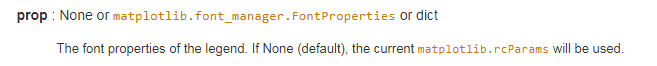一种比较简单的设置为：

font1 = {'family' : 'Times New Roman',
'weight' : 'normal',
'size'   : 23,
}

坐标轴刻度密度/间隔设置：

ax.xaxis.set_major_locator(MultipleLocator(10))

括号中的数字为对应的刻度间隔值，y轴对应类似。

坐标轴刻度值属性设置：

plt.tick_params(labelsize=23)
labels = ax.get_xticklabels() + ax.get_yticklabels()
[label.set_fontname('Times New Roman') for label in labels]

其中tick_params中可设置一系列属性，包括刻度值字体大小、方向、大小，颜色等一系列属性，具体可参见手册中关于tick_params的解释。

比较特殊的是，其中并没有对刻度值的字体进行设置的属性，所以我们需要使用下面两行进行设置，在最初使用plt.subplots中有得到一个返回值ax，我们使用ax.get_xticklabels()以及ax.get_yticklabels()来得到所有的刻度值，并使用set_fontname函数来设置属性。

坐标轴名称以及对应字体属性设置：

plt.xlabel('round',font2)
plt.ylabel('value',font2)

这种比较简单，第一个参数为坐标轴名称，第二个参数也是一个字典参数，和上文提及的dict font1格式相同。

有时候，因为调整了坐标刻度的字体大小，影响了坐标轴label的显示。所以我们需要通过调整坐标轴边距来显示label

plt.subplots_adjust(left = 0.15,bottom=0.128)

下面我们给出一个比较简单的画图过程:

#--coding:utf-8--
import  matplotlib.pyplot as plt

#数据设置
x1 =[0,5000,10000, 15000, 20000, 25000, 30000, 35000, 40000, 45000, 50000, 55000];
y1=[0, 223, 488, 673, 870, 1027, 1193, 1407, 1609, 1791, 2113, 2388];

x2 =[0,5000,10000, 15000, 20000, 25000, 30000, 35000, 40000, 45000, 50000, 55000];
y2=[0, 214, 445, 627, 800, 956, 1090, 1281, 1489, 1625, 1896, 2151];

#设置输出的图片大小
figsize = 11,9
figure, ax = plt.subplots(figsize=figsize)

#在同一幅图片上画两条折线
A,=plt.plot(x1,y1,'-r',label='A',linewidth=5.0)
B,=plt.plot(x2,y2,'b-.',label='B',linewidth=5.0)

#设置图例并且设置图例的字体及大小
font1 = {'family' : 'Times New Roman',
'weight' : 'normal',
'size'   : 23,
}
legend = plt.legend(handles=[A,B],prop=font1)

#设置坐标刻度值的大小以及刻度值的字体
plt.tick_params(labelsize=23)
labels = ax.get_xticklabels() + ax.get_yticklabels()
[label.set_fontname('Times New Roman') for label in labels]

#设置横纵坐标的名称以及对应字体格式
font2 = {'family' : 'Times New Roman',
'weight' : 'normal',
'size'   : 30,
}
plt.xlabel('round',font2)
plt.ylabel('value',font2)

#将文件保存至文件中并且画出图
plt.savefig('figure.eps')
plt.show()

最终生成的图片效果如下：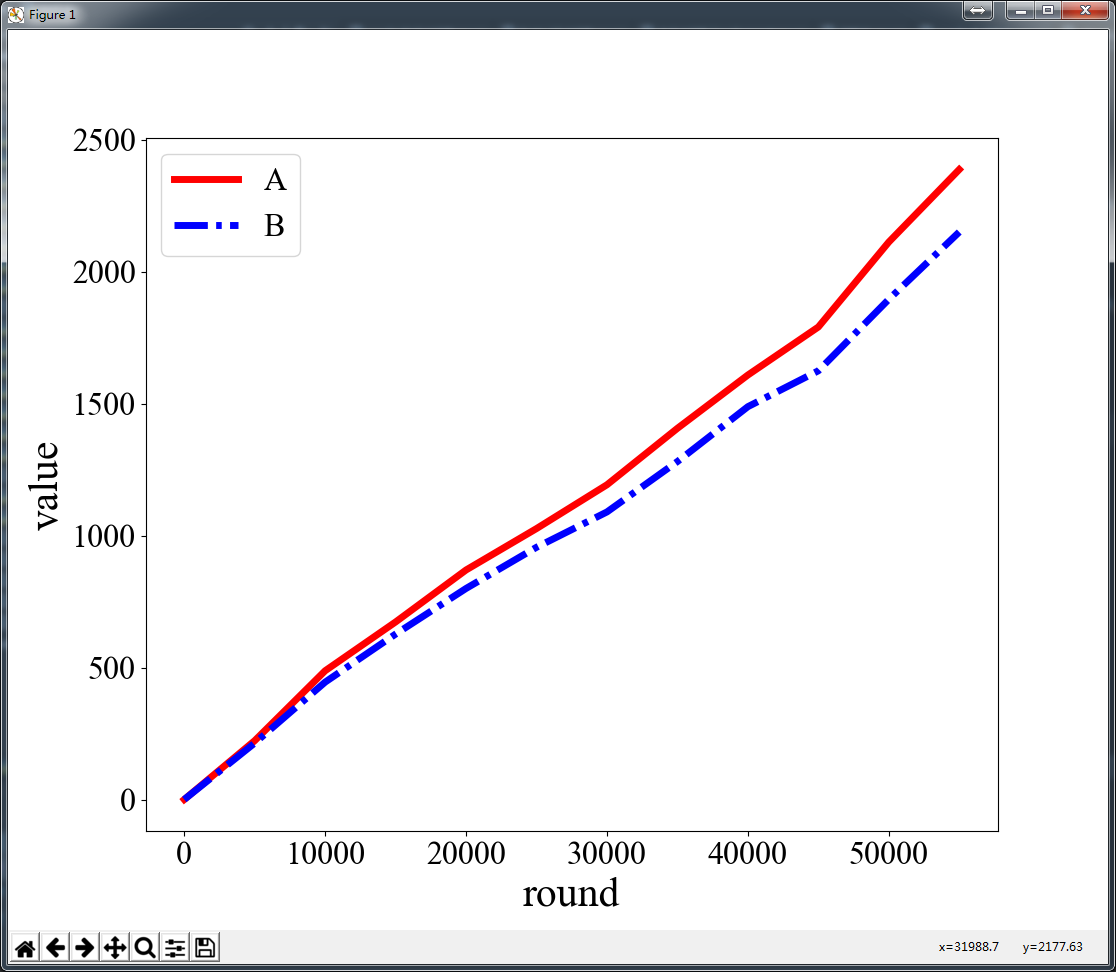更多的画图代码参考也可见手册中的Examples using matplotlib.pyplot.plot，可以翻到超链接跳转页面的最下方，有很多画图的示例，点进去即为对应实现代码。可以找到适用的代码实现方式。pyplot.subplots下也有很多很好的示例！

展开全文matplotlib
• 使用matplotlib.pyplot画带色标(colorbar)的图时候，可以设置x,y轴坐标字体的大小，但没有办法设置图例刻度条字体的大小。期望效果如下如所示。 2. 解决方案 删除seaborn中默认的条带，设置cbar=False。通过新建...
python使用seaborn画热力图中设置colorbar图例刻度字体大小
文章目录python使用seaborn画热力图中设置colorbar图例刻度字体大小1. 问题描述2. 解决方案3. 效果4. 参考

1. 问题描述
使用matplotlib.pyplot画带色标(colorbar)的图时候，可以设置x,y轴坐标字体的大小，但没有办法设置图例刻度条字体的大小。期望效果如下如所示。2. 解决方案
删除seaborn中默认的条带，设置cbar=False。通过新建一个刻度条，设置其刻度字体。
# 设置字体格式
plt.rc('font', family='Times New Roman')
plt.rcParams["font.weight"] = "bold"
plt.rcParams["axes.labelweight"] = "bold"
# 绘制热力图
h = sns.heatmap(
data=wind_array, # 指定绘图数据
cmap='YlGnBu', # 指定填充色'PuBuGn'，，jet
# center=1,
linewidths=.1, # 设置每个单元格边框的宽度
annot=False,  # 显示数值
cbar=False,
# fmt='.3f',# 以科学计算法显示数据
vmax=0.6,
vmin=0,
xticklabels=('1', '','','4','', '','7','','','10'),
yticklabels=('1', '','','4','', '','7','','','10'),
)
# ------------设置颜色条刻度字体的大小-------------------------#
cb = h.figure.colorbar(h.collections) #显示colorbar
cb.ax.tick_params(labelsize=28)  # 设置colorbar刻度字体大小。

# 添加标题, fontweight='bold'
plt.title('AAA', fontsize=35) # 'WS(m/s)'  u'T(°C)'  'P(mbar)'
plt.xticks(fontsize=28)
plt.yticks(fontsize=28)
plt.xlabel('longitude', fontsize=34)  # 经度
plt.ylabel('latitude', fontsize=34)   # 纬度
plt.rcParams['savefig.dpi'] = 600
plt.tight_layout()
plt.savefig('D:\\Data\\aaa')

# 显示图形
plt.show()

3. 效果4. 参考
https://blog.csdn.net/weixin_43718675/article/details/89451587


展开全文数据可视化
• 使用Python+matplotlib绘图进行可视化，在图形中创建轴域并设置轴域位置和大小，同时演示设置坐标轴标签和图例位置用法。 参考代码： 运行结果： 例2 绘制正线余弦图像，然后设置图例字体、标题、位置、...前言

本文的文字及图片来源于网络,仅供学习、交流使用,不具有任何商业用途,版权归原作者所有,如有问题请及时联系我们以作处理。

例1

使用Python+matplotlib绘图进行可视化，在图形中创建轴域并设置轴域的位置和大小，同时演示设置坐标轴标签和图例位置的用法。

参考代码：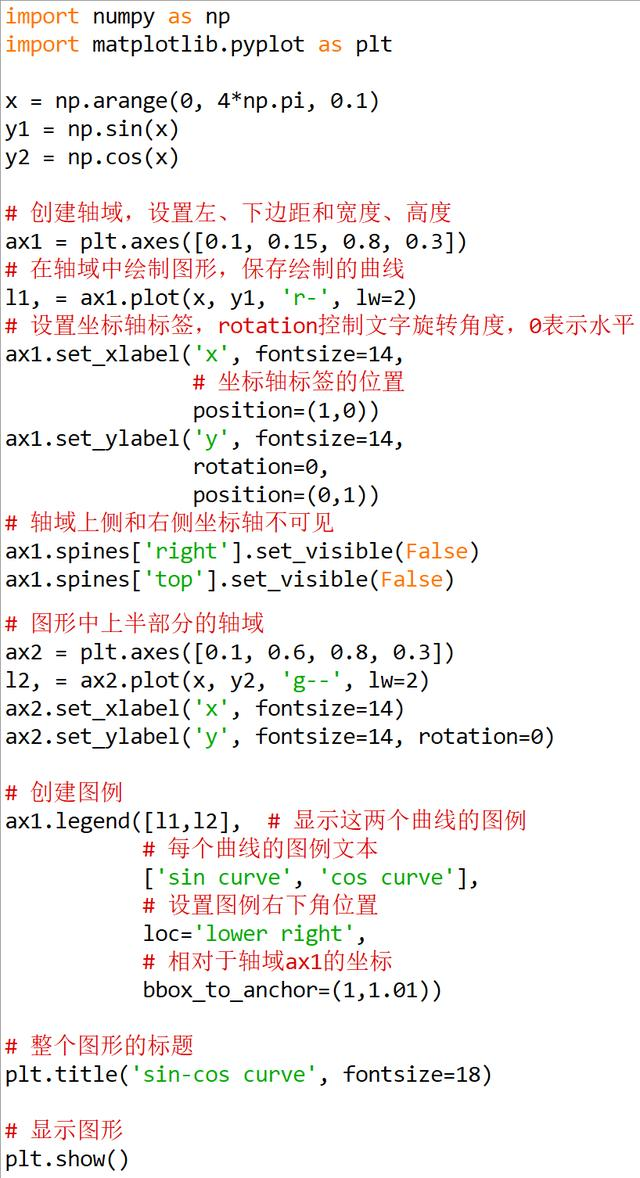运行结果：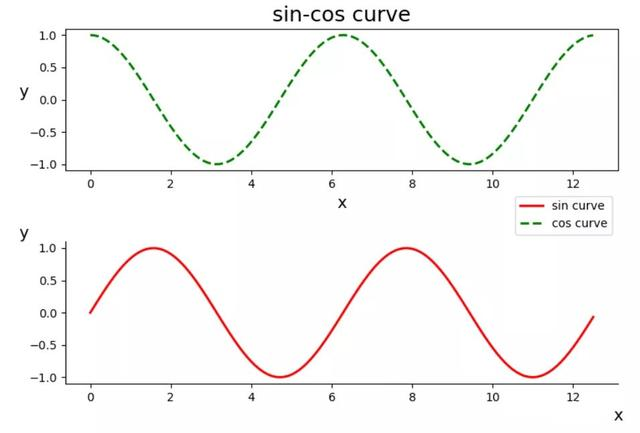例2

绘制正线余弦图像，然后设置图例字体、标题、位置、阴影、背景色、边框颜色、分栏、符号位置等属性。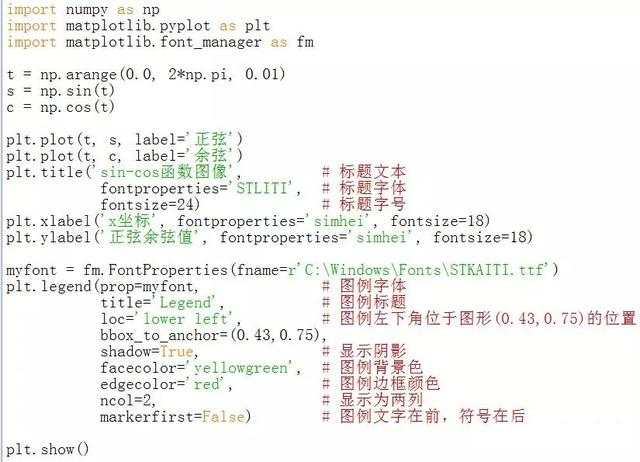运行效果：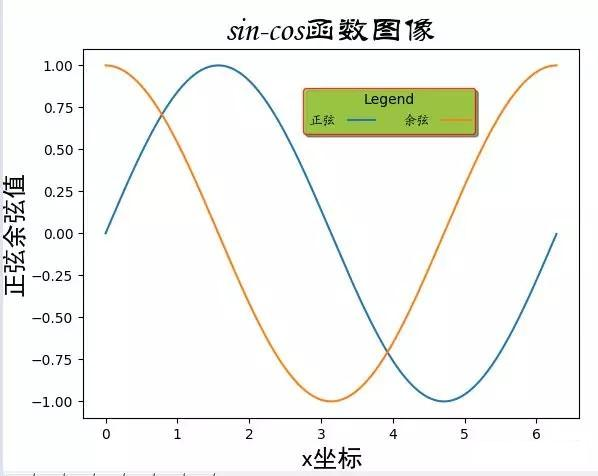例3

生成模拟数据，创建两个子图，分别绘制正弦曲线和余弦曲线，把两个子图的图例显示在一起，并显示于子图之外。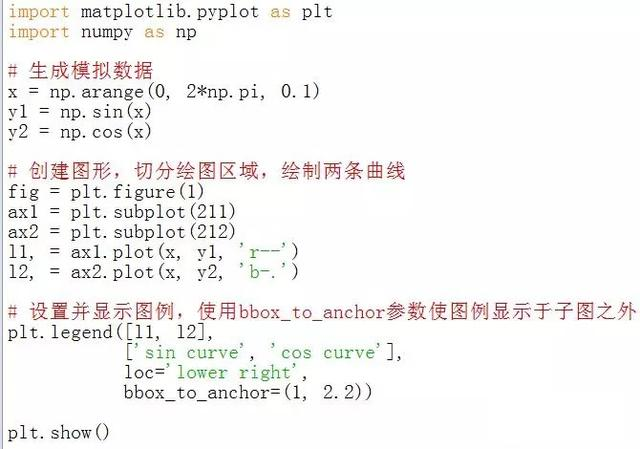运行效果：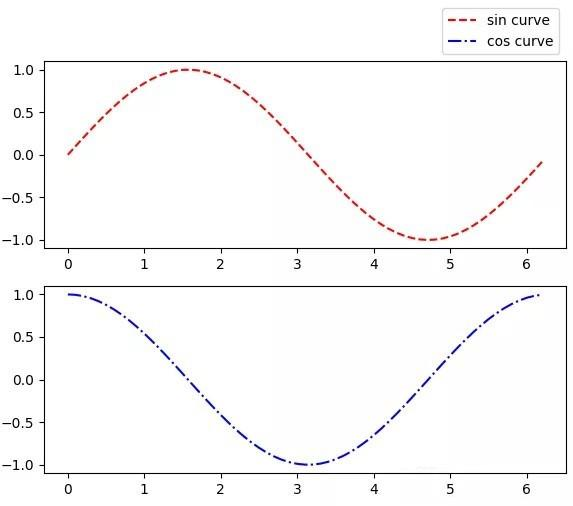例4

生成模拟数据，绘制正弦曲线、余弦曲线和两个散点图，然后分别为曲线和散点图设置图例，在一个图形上显示两个图例。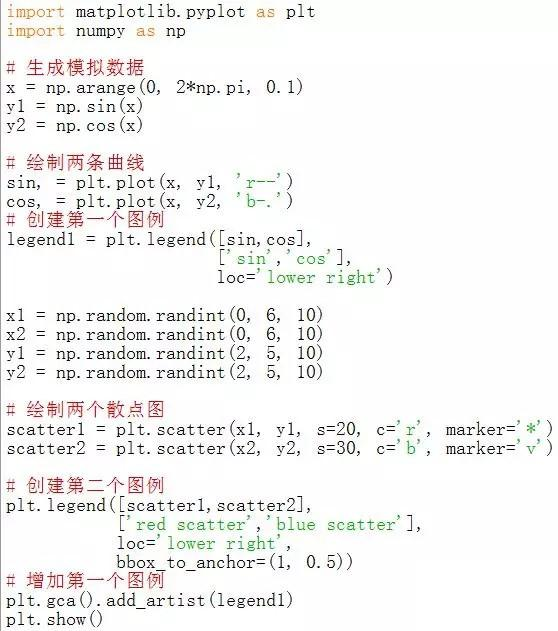运行效果：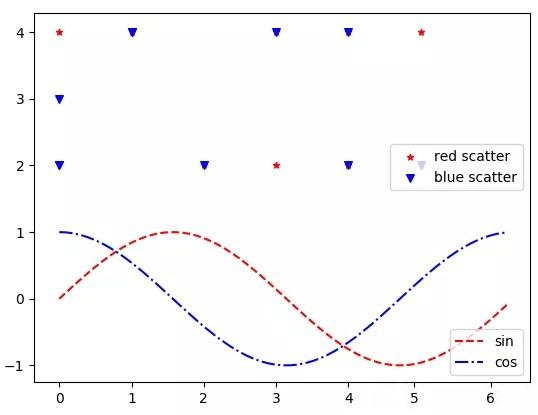欢迎点击左上角关注小编，除了分享技术文章之外还有很多福利，私信学习资料可以领取包括不限于Python实战演练、PDF电子文档、面试集锦、学习资料等。


展开全文数据可视化 可视化 数据分析 编程语言
• python 图形绘制一、图形绘制1.1简单图形1.2 改线粗、颜色、添加图例、点形状1.3 颜色设置模块1.4图例设置1.4.1 图例背景\位置\大小1.4.1.1 图例位置1.4.1.2 图例大小1.5 线类型1.6 点形状(marker)1.5 在图中添加...
• 需要两个端点来表示线，所以是两个点），matplotlib.pyplot.scatter(x,y,'.')画出的散点图中图例是三个点（这个我理解不了为什么，scatter散点的大小可以自己设置，我猜可能跟这个有关）。我画两个例子，大家看看。 ...legend
• 如下所示： ...# 设置图例字体大小 以上这篇在python plt图表中文字大小调节方法就是小编分享给大家全部内容了，希望能给大家一个参考，也希望大家多多支持软件开发网。 您可能感兴趣文章:python
• 我们会说明如何设置图中：曲线拐点形状的大小，横纵坐标刻度、标签的大小图例中形状的大小 比如要画下面这张图： 代码如下： import numpy as np import pandas as pd import matplotlib.pyplot as plt from...
• 一张字体调整好示例图： 字体大小就是 fontsize 参数 import matplotlib.pyplot as plt # 代码中“...”代表省略其他参数 ...# 设置图例字体大小 ax.legend(..., fontsize=20) 实战： import matplotlib.
• 目标：使用python的matplotlib画柱状图，以及matplotlib中文显示乱码问题。 画柱状c #导入所需要库 import matplotlib.pyplot as plt #要设置下面两行才能显示中文 Arial Unicode MS 为字体 plt.rcParams...
• python绘图时相关设置 #图的大小 plt.figure(figsize=(24,8)) #图的线宽、颜色、线类型、标签 plt.plot(x, linewidth=3.0, ...# 设置图例并且设置图例的字体及大小 font1 = {'family': 'Times New Roman', 'weig.
• 使用Python+matplotlib绘图进行可视化，在图形中创建轴域并设置轴域位置和大小，同时演示设置坐标轴标签和图例位置用法。 参考代码： 运行结果： 温馨提示 关注本公众号“Python小屋”，...可视化 数据可视化
• 1、设置刻度、图例和坐标标签字体大小 1）、设置刻度大小 plt.xticks(fontsize=20) plt.yticks(fontsize=20) 2）、设置坐标大小 ...3）、设置图例字体大小 plt.legend(..., fontsize=20) 2、添加注释（a...数据可视化
• 使用Python+matplotlib绘图进行可视化，在图形中创建轴域并设置轴域位置和大小，同时演示设置坐标轴标签和图例位置用法。 参考代码： 运行结果： 例2 绘制正线余弦图像，然后设置图例字体、标题、位置、阴影、...
• 文章目录前言黑边渐变色多线型设置双纵坐标轴去掉边框调整图例大小位置列数子图 前言 在发论文时候，常规图往往无法满足论文要求，需要进行各种特殊处理，下面展示一些处理例子。 黑边渐变色 有些杂志期刊是...
• 目录示例数据准备模块导入基础数据行/列切换单列单行格式优化调整图块形状调整图片大小旋转轴上文字方向和改变轴字体大小调整图例为横向并缩小图例增加数字标签设置图例范围加标题换颜色其他 示例 热力图一般是矩阵...数据分析
• axex: 设置坐标轴边界和表面颜色、坐标刻度值大小和网格显示 backend: 设置目标暑促TkAgg和GTKAgg figure: 控制dpi、边界颜色、...legend: 设置图例和其中文本显示 line: 设置线条（颜色、线型、宽度等）
• 【课程3.2】 图表基本元素 图表内基本参数设置 1.图名，图例，轴标签，轴边界，轴刻度，轴刻度标签等 ...# figsize：创建图表窗口，设置窗口大小 # 创建图表对象，并赋值与fig plt.title('Interes...
• 继续继续来、如题目、对文章学习后...本文翻译自Jupyter nbviewer中第四讲，主要介绍了绘制2D图像相关信息，图像位置、大小，曲线样式、宽度，坐标轴刻度、数值、标签，以及图例、标题参数的设置，还包括各量化交易
• 据我了解，大部分人在对matplotlib接触不深时都是边画图边百度，诸如这类问题，我想大家都似曾相识：Python如何画散点图，matplotlib怎么将坐标轴标签旋转45度，怎么设置图例字体大小等等。无论针对哪一个问题，...
• 使用Python+matplotlib绘图进行可视化，在图形中创建轴域并设置轴域并设置轴域位置和大小，同时演示位置坐标轴标签和图例位置用法。 首先需要安装numpy和matplotlib库,打开终端输入: pip install matplotlib -...
• set(hl,'fontsize',fs) %设置图例大小 grid on %加网格 title('普朗克黑体单色辐射能力与波长关系','fontsize',fs)%标题 xlabel('波长\it\lambda\rm/\mum','fontsize',fs)%横坐标 yl='单色辐射能力\itM\rm(\it...云计算
• 在b站看一个视频教程，边学边记笔记。...# 配置参数 # figure:控制dpi，边界颜色，图形大小，和子区(subplot)设置 # font:字体集(font family)，字体大小和样式设置 ...# legend:设置图例和其中文本显示 # line...matplotlib
• 对比下面两幅图的图例 plot(x, sin(x)) xlabel('radians') # 可以设置字体大小 ylabel('amplitude', fontsize='large') title('Sin(x)') grid() legend(['sine']) plot(x, sin(x)) xlabel('radians')...
• 目录 figure subplot 直方图：hist 散点图：scatter ...设置图片大小 调整X或者Y轴上刻度 设置中文显示 给图像添加描述信息 自定义绘制图形风格 为每条线添加图例 Matplotlib 是...
• matplotlib入门1.1 %matplotlib inline与%matplotlib notebook1.2 图片与子图1.2.1 调整子图间的间距1.2.2 设置的大小1.3 设置绘图范围1.4 设置标题1.5 设置轴标签1.6 设置刻度以及刻度标签1.7 批量设置绘图属性...数据可视化 可视化# python设置图例的大小python 订阅